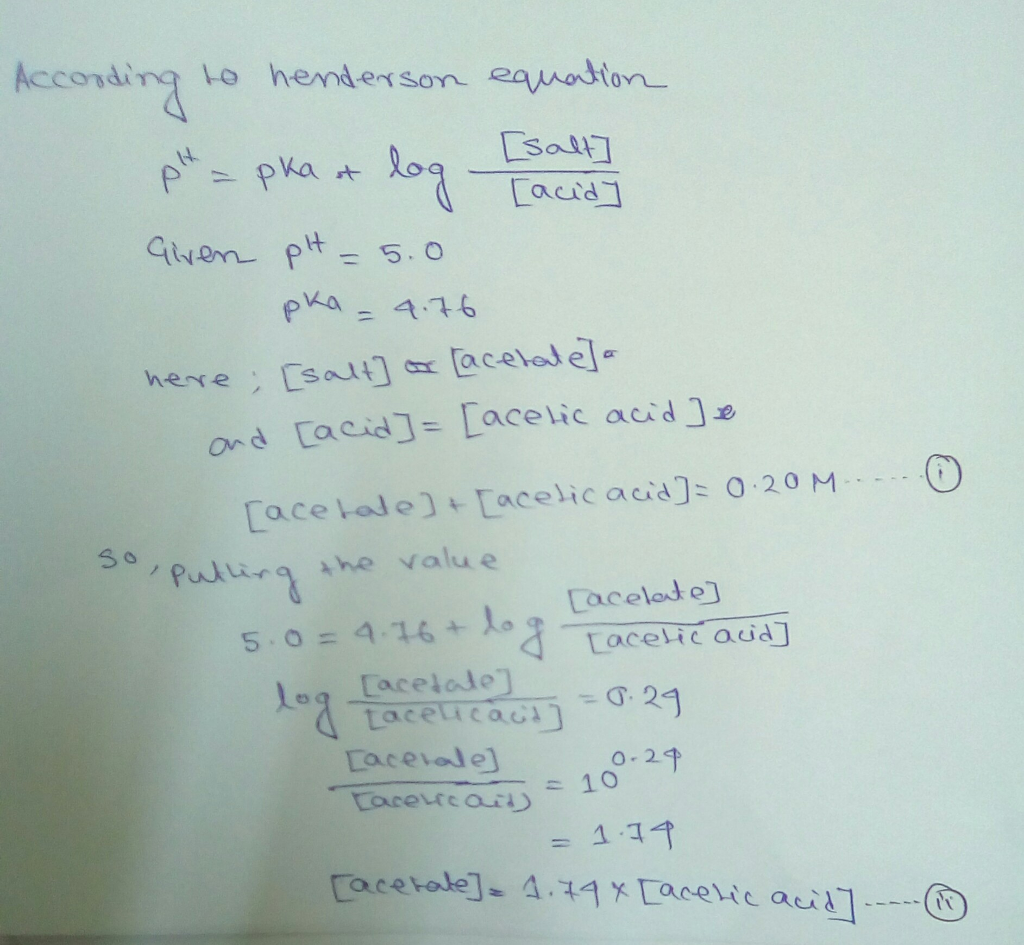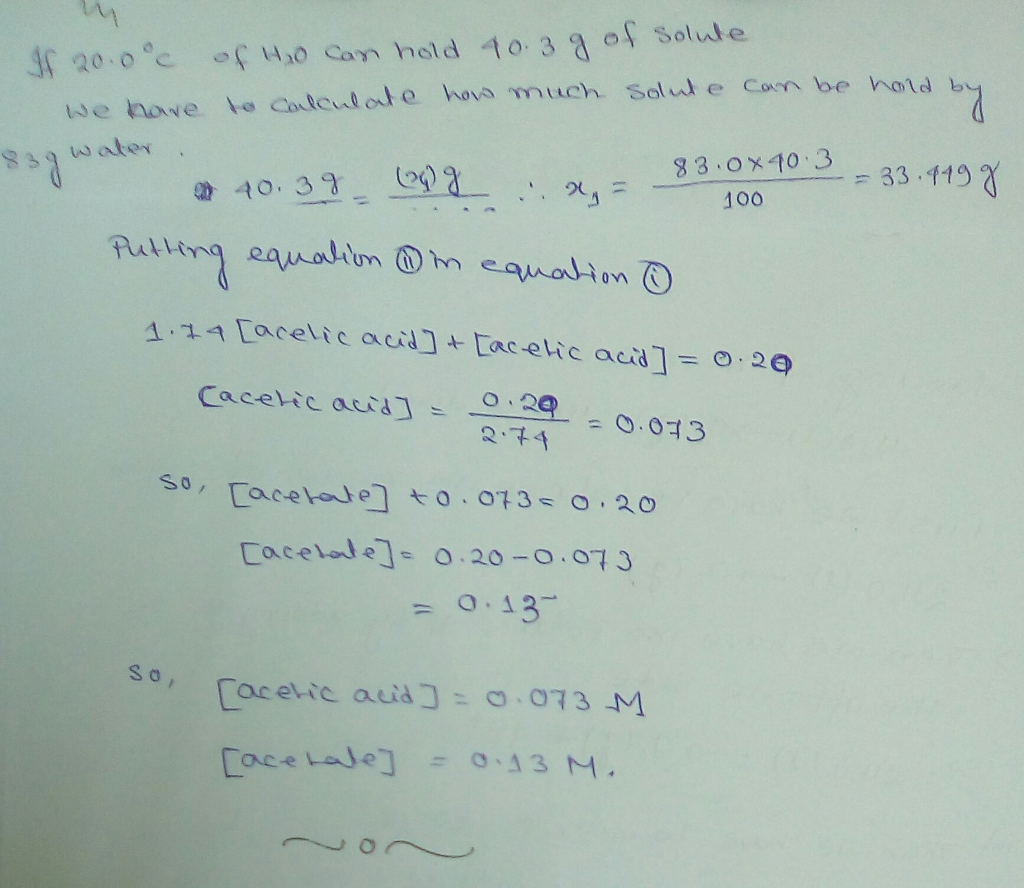# Question & Answer: What are the concentrations of acetic acid (pK_a = 4.76) and acetate in a buffer solution of…..

What are the concentrations of acetic acid (pK_a = 4.76) and acetate in a buffer solution of 0.20 M at pH 5.0? Enter your answer to two significant figures. (For example, 0.0090 and 0.0097 each have two significant figures, whereas 0.009 has only one significant figure.) [acetic acid] = M [acetate] = M